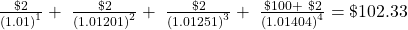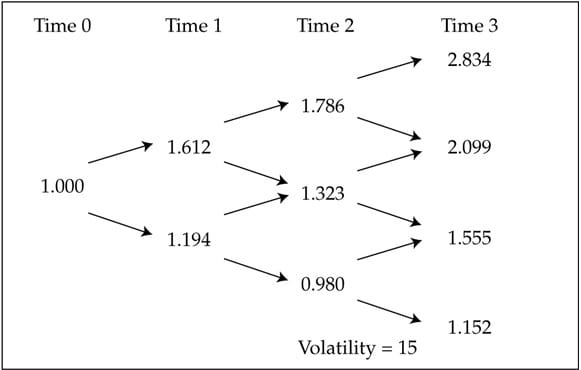Essential Concept 66: Confirming the Arbitrage-Free Value of a Bond | IFT World
101 Concepts for the Level I Exam

# Essential Concept 66: Confirming the Arbitrage-Free Value of a BondTo value an option free bond, we can either use the sport rates or a binomial tree. Since both the methods are arbitrage free, the two values should be the same.

Consider an option-free bond with four years remaining to maturity, a coupon rate of 2%, and a par value of \$100. Assume spot rates are as shown in Exhibit 3.

 Maturity (Years) One-Year Spot Rate 1 1.000% 2 1.201% 3 1.251% 4 1.404% 5 1.819%

Then the bond value can be calculated as:Next consider a binomial interest rate tree calibrated to the same spot curve (Exhibit 13).We can then use the backward induction discounting process to obtain the bond value.

 Time 0 Time 1 Time 2 Time 3 Time 4 102.3254 102.6769 101.7639 101.1892 102 104.0204 102.8360 101.9027 102 103.6417 102.4380 102 102.8382 102 102

The tree produces the same value for the bond as the spot rates and is therefore consistent with our standard valuation model.